### Home > PC > Chapter 1 > Lesson 1.2.2 > Problem1-80

1-80.

The function f(x) is shown below right. Below are functions g(x) and h(x) which are transformations of f(x). Find expressions for g(x) and h(x) in terms of f(x). Try this using an eTool. Homework Help ✎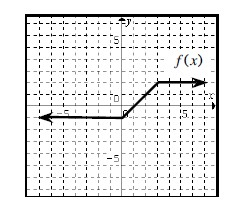a.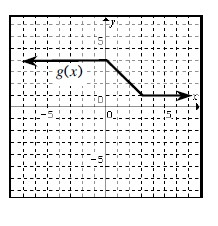b.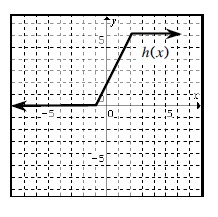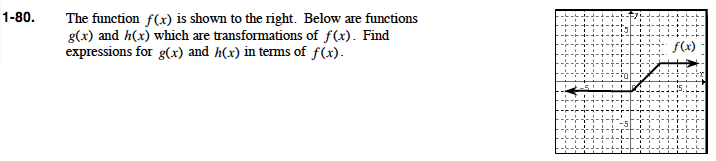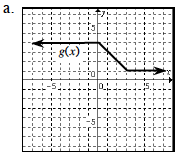Describe how the graph is reflected.

Reflected vertically: -f(x)

Describe the vertical shift.

Shifted up 3 units: f(x) + 3
g(x) = -f(x) + 3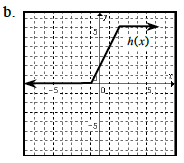Describe the horizontal shift.

Shifted left 1 unit: f(x + 1)

Describe the vertical shift.

Shifted up 1 unit: f(x) + 1

Describe the stretch factor.

Doubled: 2(f(x))
h(x) = 2f(x + 1) + 2